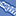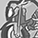# New to Qlik Sense

If you’re new to Qlik Sense, start with this Discussion Board and get up-to-speed quickly.

cancel
Showing results for
Did you mean:Creator II

## Set analysis with variables as measures

Hello,

I have to do a set analysis . The format is like below.

Sum({<FM= {MSL}, [Task Name] = {EExternal SL Engagment - SL interaction}>}[\$(vTotalyrshours)])/Sum({<FM= {ML}>}[\$(vTotalyrshours)])

\$(vTotalyrshours) is sum(Q1hours)+sum(Q2hours)+sum(Q3hours). Please suggest how to do it .  I am not getting any values in it.

Thanks,

Niha

1 Solution

Accepted SolutionsCreator II

Try this

Pretending MSL and ML are texts not  fields

=(sum({<FM= {'MSL'}, [Task Name] = {"\$(=(EExternal SL Engagment - SL interaction))"}>}Q1hours)+sum({<FM= {'MSL'}, [Task Name] = {"\$(=(EExternal SL Engagment - SL interaction))"}>}Q2hours)+sum({<FM= {'MSL'}, [Task Name] = {"\$(=(EExternal SL Engagment - SL interaction))"}>}Q3hours))

/

(sum({<FM= {'ML'}>}Q1hours)+sum({<FM= {'ML'}>}Q2hours)+sum({<FM= {'ML'}>}Q3hours))

2 RepliesCreator II

Try this

Pretending MSL and ML are texts not  fields

=(sum({<FM= {'MSL'}, [Task Name] = {"\$(=(EExternal SL Engagment - SL interaction))"}>}Q1hours)+sum({<FM= {'MSL'}, [Task Name] = {"\$(=(EExternal SL Engagment - SL interaction))"}>}Q2hours)+sum({<FM= {'MSL'}, [Task Name] = {"\$(=(EExternal SL Engagment - SL interaction))"}>}Q3hours))

/

(sum({<FM= {'ML'}>}Q1hours)+sum({<FM= {'ML'}>}Q2hours)+sum({<FM= {'ML'}>}Q3hours))Creator II
Author

HI,

Thanks.  Sorry my typo error. MSL only and all are value of a column .  I followed your suggestion the codes looks like below. It is working

=(sum({<FM ={'MSL'}, [Task Name] = {'EExternal SL Engagment - SL interaction'}>}Q1hours)+sum({< FM ={'MSL'},
[Task Name] = {'EExternal SL Engagment - SL interaction'}>}Q2hours)+ sum({<FM ={'MSL'},[Task Name] = {'EExternal SL Engagment - SL interaction'}>}Q3hours))
/
(sum({<FM ={'MSL'}>}Q1hours)+sum({< FM ={'MSL'}>}Q2hours)+sum({<FM ={'MSL'}>}Q3hours))

But I was wondering instead of trying for all quarters if it is possible to do by a variables. but I think it is not a good way. Thanks.

Niha